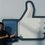# When math meets computer science: Evaluate the following Integral

This is a tricky problem that seems to defy wolfram alpha, most/all of the commercially available numerical integrators on the market, and even my trusty TI-84.

Evaluate $\int_{-\pi}^{\pi} \left(\frac{x}{\pi}\right)^{100001}\sin(x) dx$ and in general, write an algorithm that can compute $S_n = \int_{-\pi}^{\pi} \left(\frac{x}{\pi}\right)^{n}\sin(x) dx$ to at least 10 digits of accuracy in linear time.

Hint: $S_n \le 2$, why?Note by Lee Gao
7 years, 5 months ago

This discussion board is a place to discuss our Daily Challenges and the math and science related to those challenges. Explanations are more than just a solution — they should explain the steps and thinking strategies that you used to obtain the solution. Comments should further the discussion of math and science.

When posting on Brilliant:

• Use the emojis to react to an explanation, whether you're congratulating a job well done , or just really confused .
• Ask specific questions about the challenge or the steps in somebody's explanation. Well-posed questions can add a lot to the discussion, but posting "I don't understand!" doesn't help anyone.
• Try to contribute something new to the discussion, whether it is an extension, generalization or other idea related to the challenge.

MarkdownAppears as
*italics* or _italics_ italics
**bold** or __bold__ bold
- bulleted- list
• bulleted
• list
1. numbered2. list
1. numbered
2. list
Note: you must add a full line of space before and after lists for them to show up correctly
paragraph 1paragraph 2

paragraph 1

paragraph 2

[example link](https://brilliant.org)example link
> This is a quote
This is a quote
    # I indented these lines
# 4 spaces, and now they show
# up as a code block.

print "hello world"
# I indented these lines
# 4 spaces, and now they show
# up as a code block.

print "hello world"
MathAppears as
Remember to wrap math in $$ ... $$ or $ ... $ to ensure proper formatting.
2 \times 3 $2 \times 3$
2^{34} $2^{34}$
a_{i-1} $a_{i-1}$
\frac{2}{3} $\frac{2}{3}$
\sqrt{2} $\sqrt{2}$
\sum_{i=1}^3 $\sum_{i=1}^3$
\sin \theta $\sin \theta$
\boxed{123} $\boxed{123}$

Sort by:

For $n$ even, the integral is zero. For $n$ odd, we may use the series expansion of $\sin(x)$. But faster methods might exist.

- 7 years, 5 months ago

$1.9738221879\times 10^{-9}$

aka $\frac{2\pi^2}{(n+1)(n+2)}$ for odd n

But why did you put up the spoiler? It is not hard to get the definite integral for say n = 1..10. Then not hard to get the pattern and hence the basic recurrence. Then not hard to compare to the series for $\sin\pi$ and consider the error.

- 7 years, 4 months ago

Haha, nice job: that's a first order approximation, the solution is actually $S_n = -\sum_{\Delta=1}^\infty \frac{(-1)^\Delta 2\pi^{2\Delta}}{(2\Delta)!{n\choose 2\Delta}}$

I'm assuming you mean the spoiler I posted on Chandler's post: I wanted to encourage people to derive the full series rather than the obvious pattern. While the error of your method decays w.r.t to $O(n^{-4})$, it may not be very accurate for low values of $n$.

The exercise was given more as a word of caution: an elegant solution isn't always great; especially when working in the domain of numerical algorithms, care must be taken to verify instabilities in your chosen method.

- 7 years, 4 months ago

Note that Mathematica does, in fact, handle this integral incredibly well with the right tweaks.

- 7 years, 4 months ago

python:

#import math
#def s(n):
#    if n % 2 == 0:
#        return 0
#    p = 2
#    result = 2
#    for i in range(1, (n-1)/2+1):
#        p *= (1 + n - 2*i)*(2 + n - 2*i)
#        result += (-1)**i * p / (math.pi**(i*2))
#    return result


Of course you'll have to mess with this slightly to actually use exponents as high as 100001, but you get the idea...

- 7 years, 4 months ago

Hi chandler, while the solution is correct by construction, it is unfortunately unstable numerically. For example, here's the list of values your method tabulates for just the first 100 terms: http://repl.it/P4Z, in which it claims S(99) = 1.1941919707663291e+95. For one thing, we can note that the value of the integral is bounded above by $S_n \le \int_{-\pi}^{\pi} \left(\frac{x}{\pi}\right)^n dx = \frac{2}{n+1} \le 2$ One of the difficulties faced in solving this problem is finding a stable series that can compute or approximate this integral. It can be shown via a bit of analysis (or alternatively, recasting this into a nonlinear first order recurrence relation $S_{n} = 2 - \frac{n(n-1)}{\pi^2}S_{n-2}$) that suppose $S_3$ is computed with a round off error of $\epsilon$, then in general, for odd $n$, the error in the above computed series is at around $\frac{n!}{\pi^{n-1}}\epsilon$, which grows asymptotic to $O\left(\left(\frac{n}{e\pi}\right)^n \right)$.

However you're close, and with a little bit of cleverness, you can derive a stable series from this unstable series. Hint: look at the first order non-linear recurrence, find a way to express it as a first order linear recurrence, reduce it to a different sum, and find what function it corresponds to as the truncated taylor expansion of. That will help you in transforming this series into an infinite series that is stable.

- 7 years, 4 months ago

S(2n) will be zero since the integrand is odd function. For S(2n+1), you can use integration by parts twice to get $S_{2n+1} = 2-\frac{2n(2n+1)}{\pi^2}S_{2n-1}$ From here, also using the initial condition that $S_1 = 2$ you can find all values of $S_n$

- 7 years, 1 month ago

Hi Shouvik, this is the obvious recurrence associated with the problem. However, there's an interesting phenomenon that occurs when using this recurrence. Namely, using double precision floating point arithmetic, then after around 12 iterations, this series becomes unstableunstable.

If you're interested, this was a problem I considered for an optimization problem @ Cornell University this past spring, and I wrote up my experience with the problem here.

- 7 years, 1 month ago

The link I shared is broken, the correct one is https://www.dropbox.com/s/9jc9hnl5g2w5wh7/lsq2.pdf.

- 7 years, 1 month ago